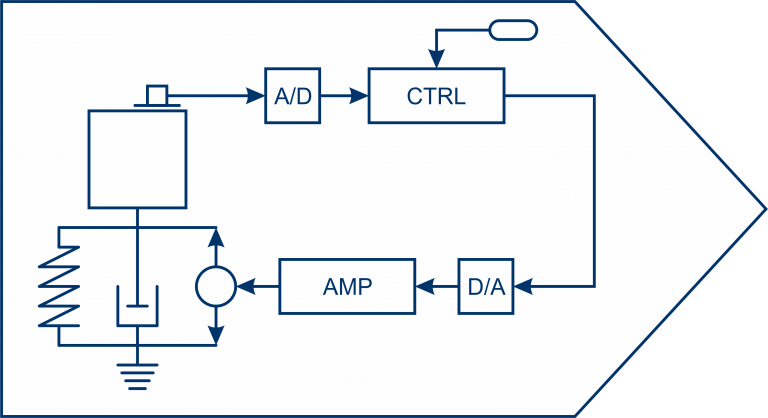Sim® Smart Structures

With AdaptroSim® Smart Structures your AdaptroSim® Structure and Vibration solutions are extended for the simulation of semi-active and active systems.Following blocks and functions from the AdaptroSim® Smart Structures can be used:

Signal Processing

Zero-OrderHold – Implements a masked version of the Simulink block ‘Zero-Order Hold’

SystemIdentificationSISO – Implements an interface which allows the system identification of a ‘ma_signalprocessing.Control’ object

PositivePositionFeedback – Is an active damping block and implements a first order positive position feedback

NbFxNLMS – Implements a narrowband SISO (Single-Input Single-Output) Filtered-reference Least-Mean-Squares algorithm (Nb FxLMS-Algorithm)

NarrowbandFeedforwardControl – Use this adaptive controller for the reduction of narrowband harmonic interference signals

IntegralForceFeedback – Is an active damping block and implements an integral force feedback

DiscreteTimeFilter – Implements a single-input single-output linear time-invariant system by means of its z-Transform

DisableExcitation – Is a simple signal feed-through block with the feature of disabling excitation depending on the parameter ‘enable_ident’

DirectVelocityFeedback – Is an active damping block and implements a direct velocity feedback

ContinuousTimeFilter – Implements a single-input single-output linear time-invariant system by means of its Laplace transform

BbFxNLMS – Implements a broadband SISO (Single-Input Single-Output) Filtered-reference Least-Mean-Squares algorithm (Bb FxLMS-Algorithm)

2ndOrderAccelerationFeedback – Is an active damping block and implements a second order acceleration feedback

2ndOrderPositivePositionFeedback – Is an active damping block and implements a second order positive position feedback

Actuators

RCShuntDetailed – Simulates the behavior of a band-limited negative capacitance

RCShuntIdeal – Simulates the ideal behavior of a capacitor with a negative capacitance value and resistor in serie

RLCShuntDetailed – Simulates the behavior of an inductor, resistor and a band-limited negative capacitance in series

RLCShuntIdeal – Simulates the ideal behavior of a series RLC shunt

RLShuntIdeal – Simulates the ideal behavior of an inductor and resistor in series

Sensors

AccelerationSensor – Implements a simple acceleration sensor

DisplacementSensor – Implements a simple displacement sensor

ForceSensor – Implements a simple force sensor

VelocitySensor – Implements a simple velocity sensor

MATLAB®

Classes

ma_signalprocessing class

ma_signalprocessing.AbstractFilter – Implements an abstract filter and passes the properties and methods to the subclasses ma_signalprocessing.FilterContinuousTime and ma_signalprocessing.FilterDiscreteTime

ma_signalprocessing.AdaptiveFIRFilterParameter – Provides a basic parameter set of an adaptive finite impulse response filter

ma_signalprocessing.Control – Provides a class for the commissioning of the adaptive Filtered-Reference Least Mean Squares (FxLMS) algorithm

ma_signalprocessing.FilterContinuousTime – Provides a class for the commissioning of the adaptive Filtered-Reference Least Mean Squares (FxLMS) algorithm

ma_signalprocessing.FilterDiscreteTime – Parameterizes and computes a discrete-time AC coupling filter

ma_signalprocessing.SystemIdentification – Provides an option interface for system identification from Simulink

Common Matlab® functions

ma_import_fft_analyser_data – Imports data table and header from ASCII

ma_fft_analyser_frf_plot – Returns the FRF, recorded by an FFT-analyser

ma_common package

ma_common.Channel – Represents a data channel (i.e. sensor) transporting system information

ma_common.ContinousTimeStateSpace – Represents a continuous-time state-space model

ma_common.DiscreteTimeStateSpace – Represents a discrete-time state-space model

ma_common.Frf – Abstract interface definition, defining frequency response function of a linear-time-invariant system

ma_common.GenericSignal – Represents a signal with its channels and units of the abscissa and ordinate

ma_common.GenericFrf – Represents the frequency response function of a system

ma_common.Geometry – Class definition for a geometry consisting of nodes and edges

ma_common.ModalSystem – Represents system dynamics described by means of modal vectors, modal frequencies and modal damping

ma_common.Node – Represents a node in a Cartesian coordinate system by its x-, y- and z-coordinates

ma_common.SecondOrderSystem – Describes a second order system using a second order linear differential equation

ma_common.Signal – Signal data container with channels that share a common abscissa

ma_common.StateSpace – Represents the time-domain state-space model of a system

ma_common.System – Represents a system with its input- and output-channels

ma_common.Unit – Represents units by exponents and factors

Compatibility Consideration

System requirements: AdaptroSim requires a Windows 64bit environment, a working copy of Matlab 2014b or later installed and you have a C compiler setup via mex -setup c command as described here.